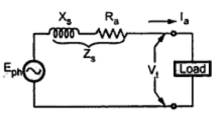Equivalent Circuit of an Alternator

From the previous discussion it is clear that in all there are three important parameters of armature winding namely armature resistance Ra, leakage reactance XL and armature reaction reactance Xar. If Eph is induced e.m.f. per phase on no load condition then on load it changes to E' due to armature reaction as shown in the equivalent circuit. As current flows through the armature, there are two voltage drops across Ra and XL as Ia Ra and respectively. Hence finally terminal voltage Vt is less than E' by the amount equal to the drops across Ra and XL.Fig. 1  Equivalent circuit

In practice, the leakage reactance XL and the armature reaction reactance Xar are combined to get synchronous reactance Xs.
Hence the equivalent circuit of an alternator gets modified as shown in the Fig. 1.Fig. 2  Equivalent circuit of an alternator

Thus in the equivalent circuit shown,
Eph = induced e.m.f. per phase on no load
Vtph = terminal voltage per phase on load
Iaph  = armature resistance per phase
Zs = synchronous impedance per phase